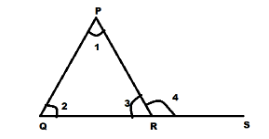QuestionAnswers

# State 'T' for true and 'F' for false.(1) In a triangle, the measure of exterior angle is equal to the sum of the measure of interior opposite angles.(2) The sum of the measures of the three angles of a triangle is ${90^ \circ }$.(3) A perpendicular is always at ${90^ \circ }$ to a given line or surface.a) T T Fb) T F Fc) T F Td) F T FVerified
128.4k+ views
Hint: Here we will check each of the given statements one by one and state them as true or false.
(1) Exterior angle is the angle between any side of a triangle, and a line extended from the next side.
So we will first construct a triangle with an exterior angle and then check whether the exterior angle comes out to be equal to the sum of two opposite interior angles by using the linear pair property
Linear pair states that the sum of two adjacent angles formed by two intersecting lines is ${180^ \circ }$.
(2) Here we will use the following formula to find the sum of all angles of the triangle.
The sum of all angles of a polygon with n number of sides is given by:-
${\text{sum of angles}} = \left( {n - 2} \right) \times {180^ \circ }$

Let us first consider the statement 1
(1) Let us construct a triangle PQR with QR extended at S and $\angle 4$ as the exterior angle.Now apply linear pair property for line QS we get:-
Since linear pair property states that the sum of two adjacent angles formed by two intersecting lines is ${180^ \circ }$.
Hence we get:-
$\angle 3 + \angle 4 = {180^ \circ }$………………………….(1)
Now we know by the angle sum property of a triangle that the sum of all angles of a triangle is ${180^ \circ }$.
Hence we get:-
$\angle 1 + \angle 2 + \angle 3 = {180^ \circ }$…………………………(2)
Equating equations 1 and 2 we get:-
$\angle 1 + \angle 2 + \angle 3 = \angle 3 + \angle 4$
Solving it further we get:-
$\angle 1 + \angle 2 = \angle 4$
Hence we get the exterior angle is equal to the sum of two opposite interior angles
Therefore, statement 1 is true.
Now let us consider the statement 2
(2) We know that the sum of all angles of a polygon with n number of sides is given by:-
${\text{sum of angles}} = \left( {n - 2} \right) \times {180^ \circ }$
Now since triangle has 3 sides therefore,
$n = 3$
Now applying the above mentioned formula we get:-
${\text{sum of angles}} = \left( {3 - 2} \right) \times {180^ \circ }$
Simplifying it further we get:-
${\text{sum of angles}} = \left( 1 \right) \times {180^ \circ } \\ \Rightarrow {\text{sum of angles}} = {180^ \circ } \\$
Therefore the sum of the measures of the three angles of a triangle is ${180^ \circ }$
Therefore, statement 2 is false.
No let us consider statement 3
(3) We know that the property of being perpendicular is the relationship between two lines which meet at a right angle.
This means that a line is said to be perpendicular to another line if the two lines intersect at a right angle (${90^ \circ }$).
Therefore, a perpendicular is always at ${90^ \circ }$to a given line or surface.
Hence statement 3 is true.
Now since we got statement 1 is true, statement 2 is false and statement 3 is true
Therefore option c is the correct option.

Note: Students should take a note that while applying the exterior angle property the angles which are opposite to the exterior angle should be considered.
The property of being perpendicular is the relationship between two lines which meet at a right angle.